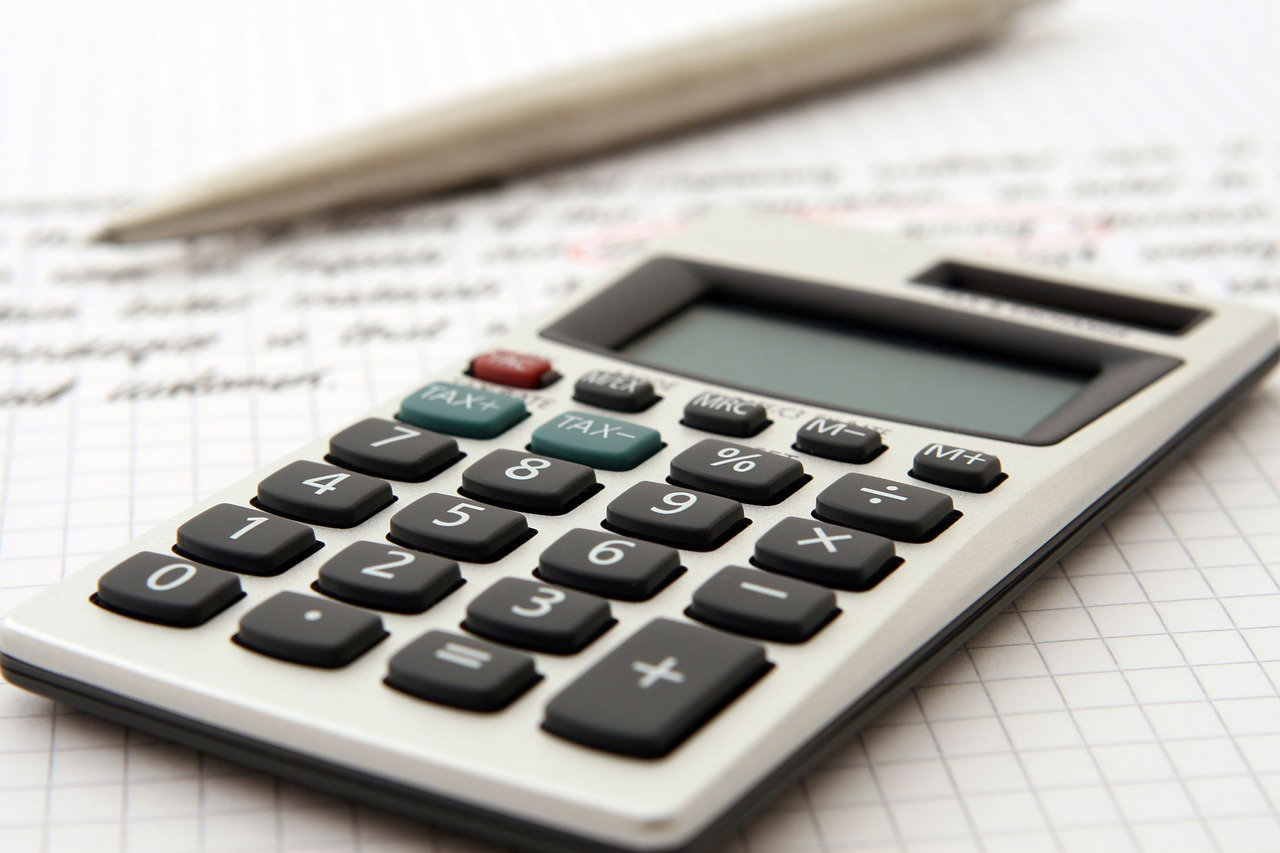What Should You Know About Preparing For Math Competitions?Math is a subject in which you need to have good skills.  Whether it is an AMC math competition or some other exam, you should have proper preparation to crack them.  Now let’s take a look at the various courses:

Geometry Preparation

If you are thinking of AMC8 and MathCounts, introductory level geometry knowledge is a must to prepare for the examination. It covers fundamentals and theorem covering points, lines, angles, area, etc. As a student, you need to study various geometric shapes like the triangles, quadrilateral, and circles and learn their basic properties.

Introductory Math Challenge

It is an initial level course for 6-8 grade students who have no experience in solving in-depth problems and are inexperienced with math competitions. You may be studying math at school level but may not be aware of the math skills to solve the problems.  In an introductory course, you will come across the contents including pre-algebra, beginning algebra, elementary geometry, basic number theory concepts, counting, and probability. You will gain skill in creative thinking, logical reasoning, analytical and problem-solving skills. You will also get exposure to various mathematics competition.

In advanced math challenge, students learn about topics like beginning algebra, basic geometry, basic and number theory concepts, counting strategies, logic, and probabilities. You will not just learn practical skills of problem-solving which will be in addition to the school curricula but will also develop skills in creative thinking and will develop skills for logical reasoning, and presentations.

Preparing for AMC 10

When you are preparing for AMC-10, you will need to have a fundamental knowledge of Algebra-1, Geometry, Basic Number Theory, Counting and Probability to the 10th-grade level. You will be studying the topics like the polynomials, inequalities, the special algebra techniques, triangles, and polygons. Other topics like collinearity and concurrency, vector and co-ordinates, number and divisibility, modular arithmetic, advanced counting strategies, the binomial coefficients, sequences, and series, etc. Then there are other topics like and advanced problem-solving techniques that are involved in math contests.

Preparing for AMC 10, 12

If you are preparing for AMC 10 and 12, then you will need to prepare for the topics like advanced number theory and combinatorics, polynomials, inequalities, special algebraic techniques and many other topics that form AMC practice problems.

Finally,

When you are preparing for AMC math competitions, these are the few things you should know!

This site was built using